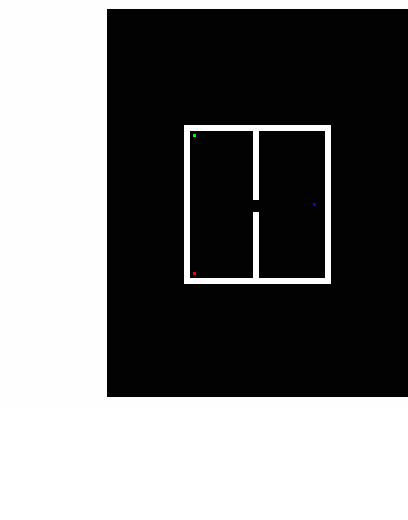Example 9. Chambered Detonation of Super-Colors

From three separate pixels, seen as real color blue, green, and red in frame #1 (9.1, below), one sees the positions of three super-color detonations.  B, G, R values are initially set to 100,000, 200, and 200, respectively, for these three separate pixels.  Diffusion then occurs.  Anything in the super-color range (i.e., greater than or equal to one for any of the three channels) is set to alternating black and gold.  The animation uses frames 1-10, 19, 20, 29, 30, 39, 40, ...199, 200.  It loops, but one can recognize frame #1 as having white walls and a white barrier with a hole in it.  As diffusion continues, the color wave fronts get diluted into the visible range, i.e., R, G, B values all less than or equal to 1.

In this simulation, blue is overwhelming:  It knocks out the entire right-side chamber, goes through the small hole in the barrier, and overcomes the diffusion of red and green in the left chamber until all real color has been converted to super-color.  There is some degradation of the images as they are packages into the animated gif for convenient viewing. The PDF link given below also has high resolution images of the entire animation.Previous examples have used a Mathematica function (ListConvolve) to run kernels over images for diffusion simulation and averaging.  The problem with standard kernel convolutions such as these is that rows and columns of pixels are lost per convolution cycle. For example, a 3 x 3 kernel causes the loss of two rows and two columns per convolution.  These are the four edge rows and columns of the image.  In this example, independent code has been written to cause pixel averaging (Fick-like) that simulates diffusion without loss of rows and columns.  This code approximates a 4 x 4 kernel with each element set to 1/16. There are two averaging operations per cycle.  The first averaging procedure uses a 2 x 2 matrix to find the mean of the four pixels it contains.  The entire image is tiled with these separate submatrices.  In the second averaging procedure per cycle, an independent set of 2x2 matrices is run over the entire image in a manner identical to the first set - except all submatrices are offset by one row and one column.  We will dub this methodology 2x2^2 averaging, read as "two by two squared" averaging.

2x2^2 averaging beginning with a pixel with a very large super-color is like a detonation; there is rapid and isotropic diffusion of super-color values down to real color within a 'shock wave' that develops at the leading edge of these groups of communicating submatrices.  This type of mathematics is discrete, whereas Fick's law (for physical diffusion processes) is given as a continuous second derivative.  Both our discrete 2x2^2 averaging and Fick lead to an equilibrium state of maximum entropy wherein all pixels or physical areas reach equally diluted values modulated only by sampling statistics in the case of Fick (e.g., photon shot noise per volume or time sampled).  In our discrete 2x2^2 analogy, there is no sampling error because we are averaging in a computer, not real space.

With the blue super-color pixel initially set to R=0, G=0, B=100,000, 2x2^2 averaging first produces four pixels of value B= 100,000/4 = 25,000 and then produces (through the staggered 2x2's) four more 2x2 areas of pixels with B=100,000/16 .  In the next cycle of averaging, this 16 pixel area contains 12 edge pixels that overlap with eight new 2x2 submatrices that in turn are averaged with staggered submatrices.  As one traces a single pathway for the detonation - along a diagonal (see 9.2 below), it is deduced that distance from the starting pixel (lower left) increases towards the upper right of the figure at a distance of 2N and with a dilution of 2^4N, where N is the cycle number.  Dashed and solid 2x2 submatrices within the figure indicate the framing and staggered framing of submatrices within each cycle.  Counting from cycle N=0, by the 4th cycle there is a leading edge of diagonal pixels at a distance of 8 pixels with a dilution factor of 2^16 = 65,536.  One more step and the initially valued (100,000) super-color becomes visible at a distance of nine pixels.  This is, in fact, observed in our simulation - given that the image size is doubled in width and height for display.Discrete 2x2^2 averaging has an advantage over Fick in that we can easily create chambers, barriers, and pores or windows by setting the range of certain convolution areas and their connectivity.  Fick requires one to formulate and solve specific differential equations for each particular situation.  Alternatively, one can simulate either type of diffusion by a random walk; however, this is computer intensive and approximate. In the example shown above, two chambers were constructed with a communicating window in an internal barrier or wall.  2x2^2 averaging is run independently in both chambers with no overlap of any submatrices.  Mixing occurs by constructing a third entity that is a mixing chamber which overlaps both large chambers by a few pixels.  The shape, size, and rules for mixing within this chamber are especially important if the overall number of pixels in the image is small.  Parenthetically, we can mention that the properties of the mixing chamber can be set to rules that do not simulate diffusion, but rather, simulate amplification or other effects.  Please see the code in nb format and PDF format for better insight.  From the code, one can see that we were careful not to increase or decrease the overall luminosity of the pixels being mixed, and that the communication between the two chambers is bi-directional and non-preferential.